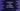# 4 ways to add the digits of a number in JavaScript## JavaScript program to add the digits of a number:

In this post, I will show you how to write a `JavaScript` program to add the digits of a given number or it will find the sum of the digits of a number. For example, if the number is `234`, it will print `9`.

### Method 1: Find the sum of digits of a number by using a `while` loop:

With this approach, we will use one `while` loop to find the sum. It will follow the following steps:

• Initialize one variable `sum` as 0 to hold the final sum.
• Run one `while` loop until the number is greater than 0.
• In the loop, get the rightmost digit of the number and add it to the `sum` variable.
• At the end of each iteration, remove the rightmost digit from the number.

The loop will stop once all the digits are removed from the number or the number become 0.

The following program shows how to write it in `JavaScript`:

``````let n = 234;
let sum = 0;

while(n){
sum += n % 10;
n = Math.floor(n/10);
}

console.log(`Sum: \${sum}`);``````
• The number is assigned to the variable `n`. The `sum` variable is initialized to 0.
• It uses the modulo operator, `%`, to get the rightmost digit of the number. The operation `n % 10` returns the remainder of the division operation `n / 10` or the rightmost digit of the number `n`.
• `Math.floor(n/10)` removes the rightmost digit of `n`. The value of `n` is updated by this value at the end of each loop iteration.

If you run this program, it will print `9`.

The above example will update the value of `n` to 0. We can initialize one new variable before the loop starts:

``````let n = 234;
let sum = 0;

let no = n;
while(no){
sum += no % 10;
no = Math.floor(no/10);
}

console.log(`Sum of digits of \${n}: \${sum}`);``````

It created a new variable `no` before the `while` loop starts. If you run this program, it will not modify the value of `n`:

``Sum of digits of 234: 9``

### Method 2: By using a `for` loop:

We can use the same approach with a `for` loop to find the sum. The syntax of `for` loop is:

``````for(initialize, condition, update){
// body
}``````
• The first step `initialize` runs once. This is used to initialize a variable.
• The second step `condition` checks for a condition. The loop will run until it is `True`.
• The third step `update` runs at the end of each iteration. This is used mainly to update a variable.

The body of the loop runs until the `condition` is `True`. We can use a `for` loop to find the sum of all digits of a number as shown below:

``````let n = 679;
let sum = 0;

for(let no = n; no > 0; no = Math.floor(no/10)){
sum += no % 10;
}

console.log(`Sum of digits of \${n}: \${sum}`);``````

• It initializes the variable `no` as `n` when the loop starts.
• At the end of each iteration of the loop, it assigns `Math.floor(no/10)` to the variable `no` i.e. it removes the last digit of the number.
• The loop runs until the value of `no` is greater than 0.
• In the body of the loop, it adds the last digit of `no` to the `sum` variable.

It will print the below result:

``Sum of digits of 679: 22``

### Method 3: By converting the number to `String`:

The number can be converted to a `String` and we can find the digits by iterating over the characters of the string. The following example shows how it works:

``````let n = 679;
let sum = 0;

String(n).split('').forEach(e => sum += Number(e));

console.log(`Sum of digits of \${n}: \${sum}`);``````

• It uses the `String()` constructor to convert the number `n` to `String`.
• It use the `split()` method to split the string to an array of digits. For example, for the number `679`, it will return the array `['6', '7', '9']`.
• The `forEach` loop iterates over the characters of the array. It converts the characters to a number with the `Number()` constructor and adds it to the `sum` variable.

### Method 4: By using the `reduce()` function:

The `reduce()` function is an array operation. It uses a reducer function on each element of the array and calculates a final result. We can use these function to find the sum of all digits of a number.

``````let n = 679;

let initialValue = 0;
let sum = String(n).split('').reduce((accumulator, currentValue) => accumulator + Number(currentValue), initialValue);

console.log(`Sum of digits of \${n}: \${sum}`);``````

• The number `n` is converted to a `String` and with the `split()` method we get an array of characters.
• The `reduce` function takes the second parameter `initialValue` as the starting value of the `accumulator`.
• On each step, it converts the current character to number and adds it to the current value of the `accumulator`. This value is passed as the `accumulator` value on next call.
It will return the sum of all digits of the number `n`.
``Sum of digits of 679: 22``